# RS Aggarwal Solutions for Class 8 Maths Chapter 10 - Profit and Loss

RS Aggarwal Solutions for Class 8 Maths Chapter 10- Profit and Loss, are provided here. Our expert faculty team has prepared solutions in order to help you with your exam preparation to acquire good marks in Maths. RS Aggarwal Solutions book for Class 8 Maths comes in very handy at this point. Our solution module utilizes various shortcut tips and practical examples to explain all the exercise questions in a simple and easily understandable language. If you wish to secure an excellent score, solving RS Aggarwal Class 8 Solutions is an utmost necessity. These solutions will help you in gaining knowledge and strong command over the subject. Practicing the textbook questions will help you in analyzing your level of preparation and understanding of the concept.

In order to help you to solve the problems, we at BYJU’S have designed these solutions in a very lucid and clear manner that helps students solve problems in the most efficient possible ways. Here is the RS Aggarwal Solutions for Class 8 Maths Chapter 10 where solutions are solved in detail. Download pdf of Class 8 Chapter 10 in their respective links.

## Download PDF of RS Aggarwal Solutions for Class 8 Maths Chapter 10 – Profit and Loss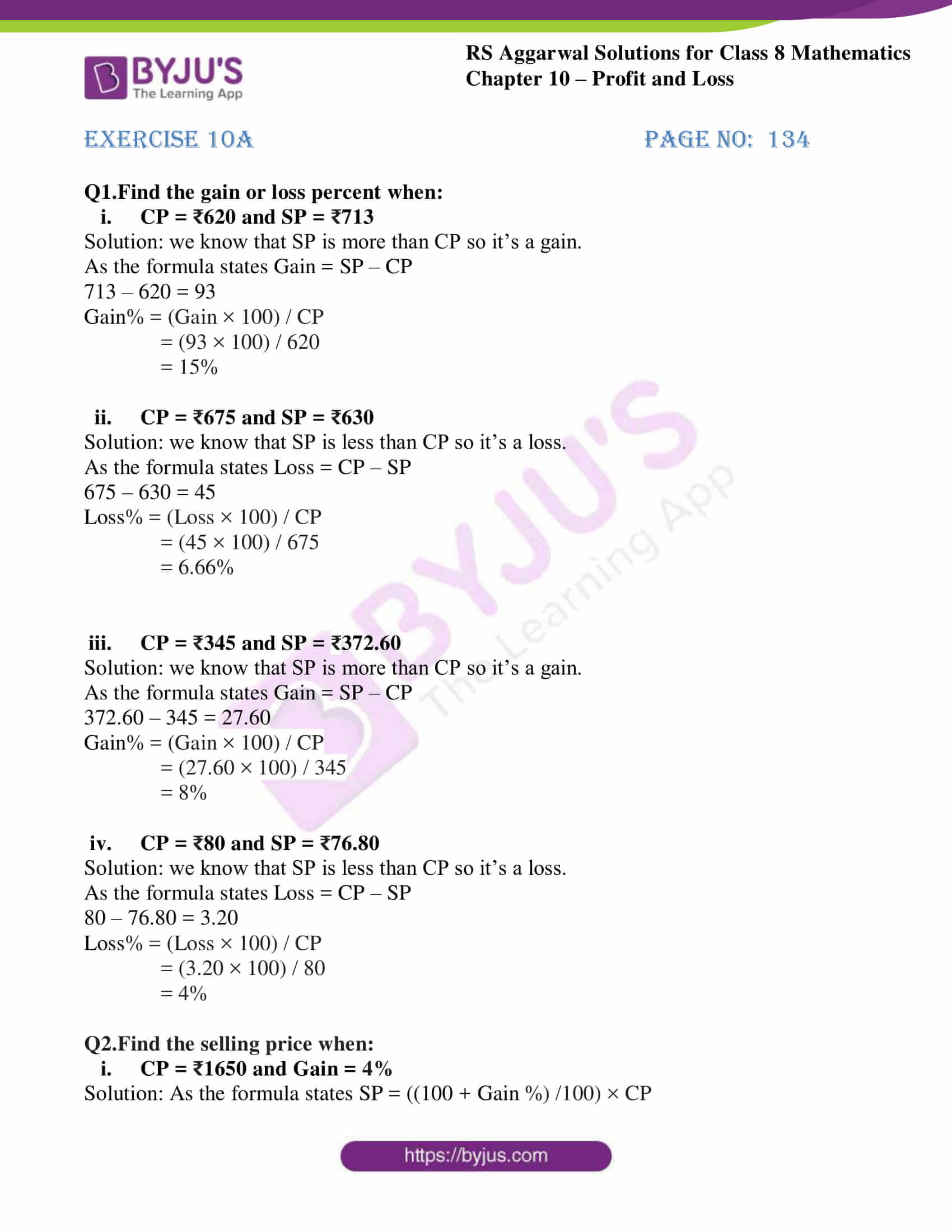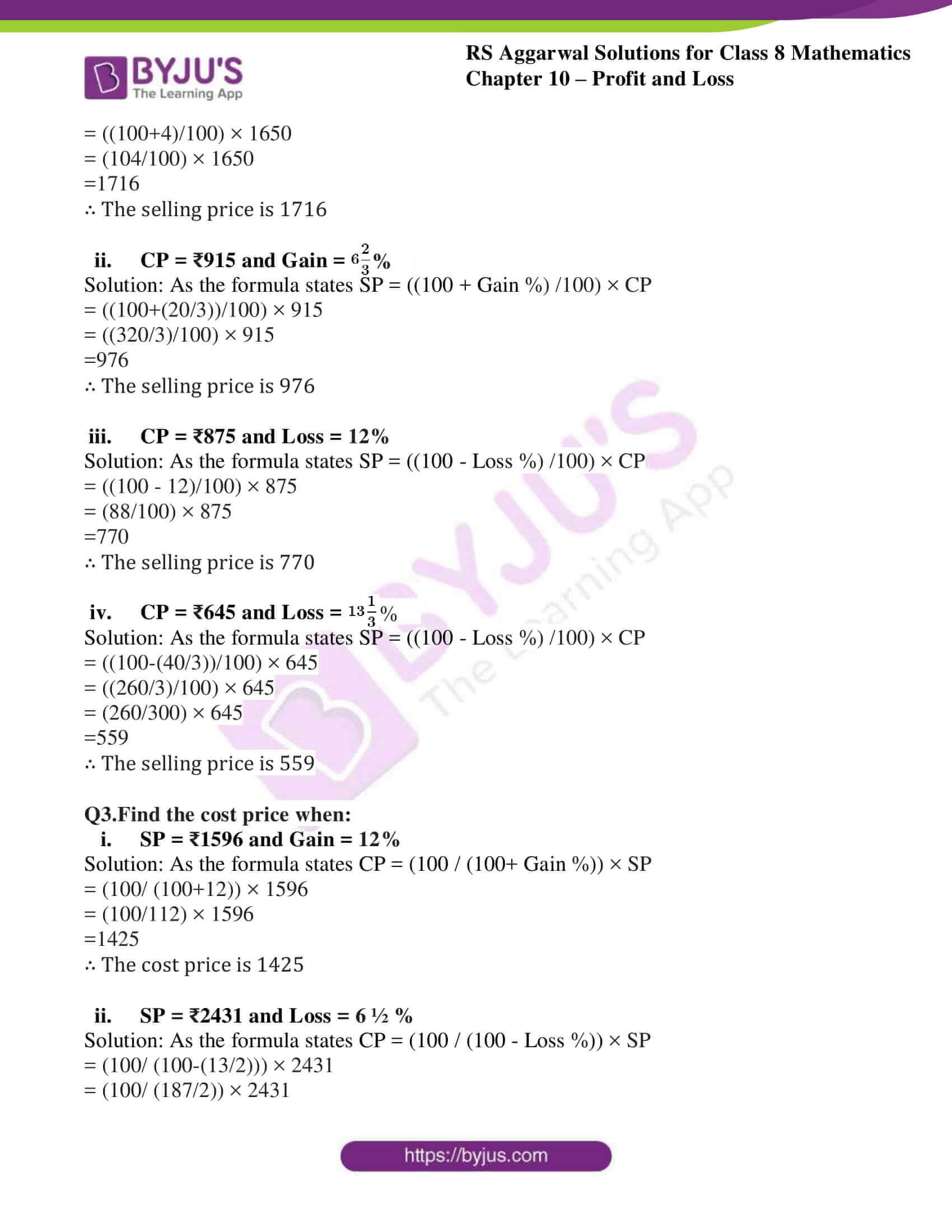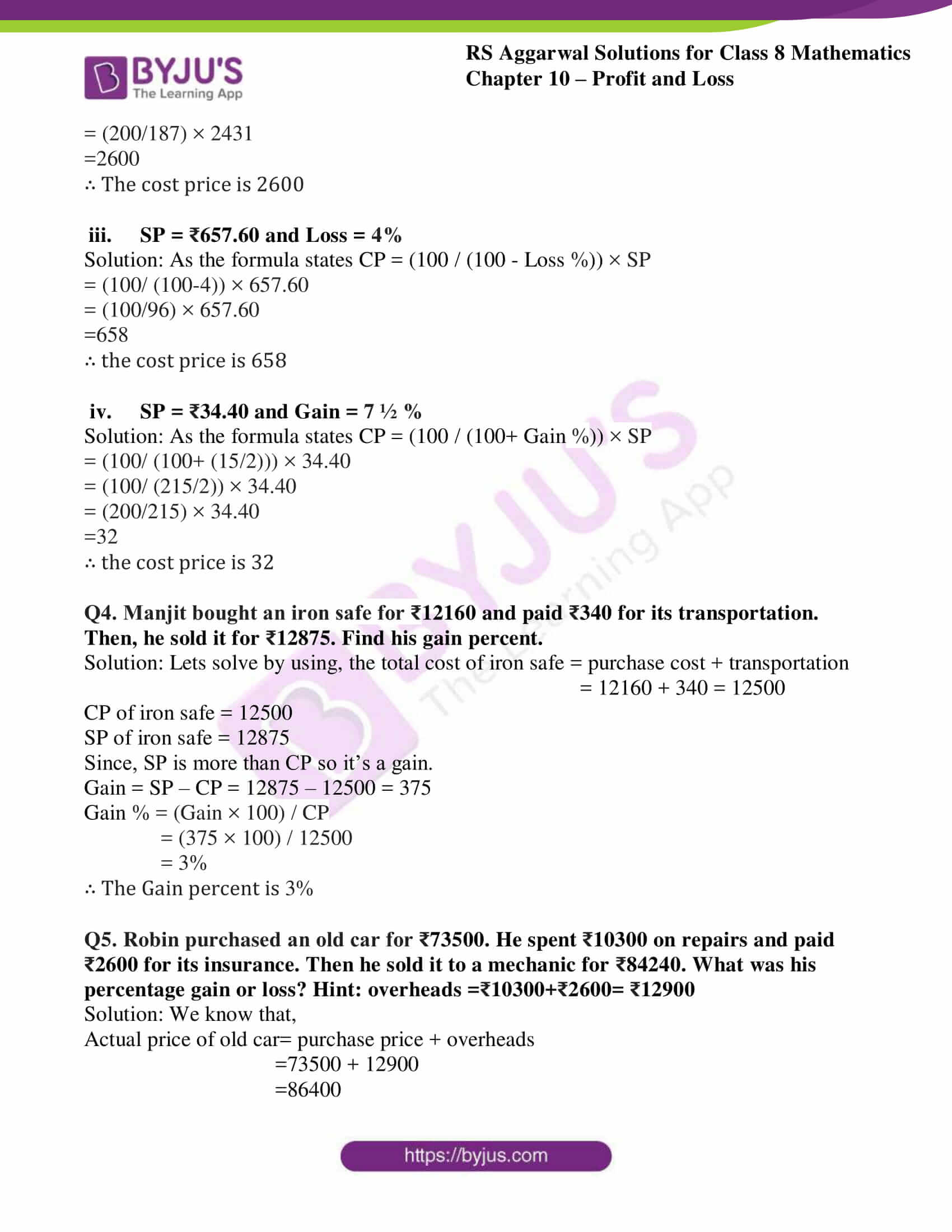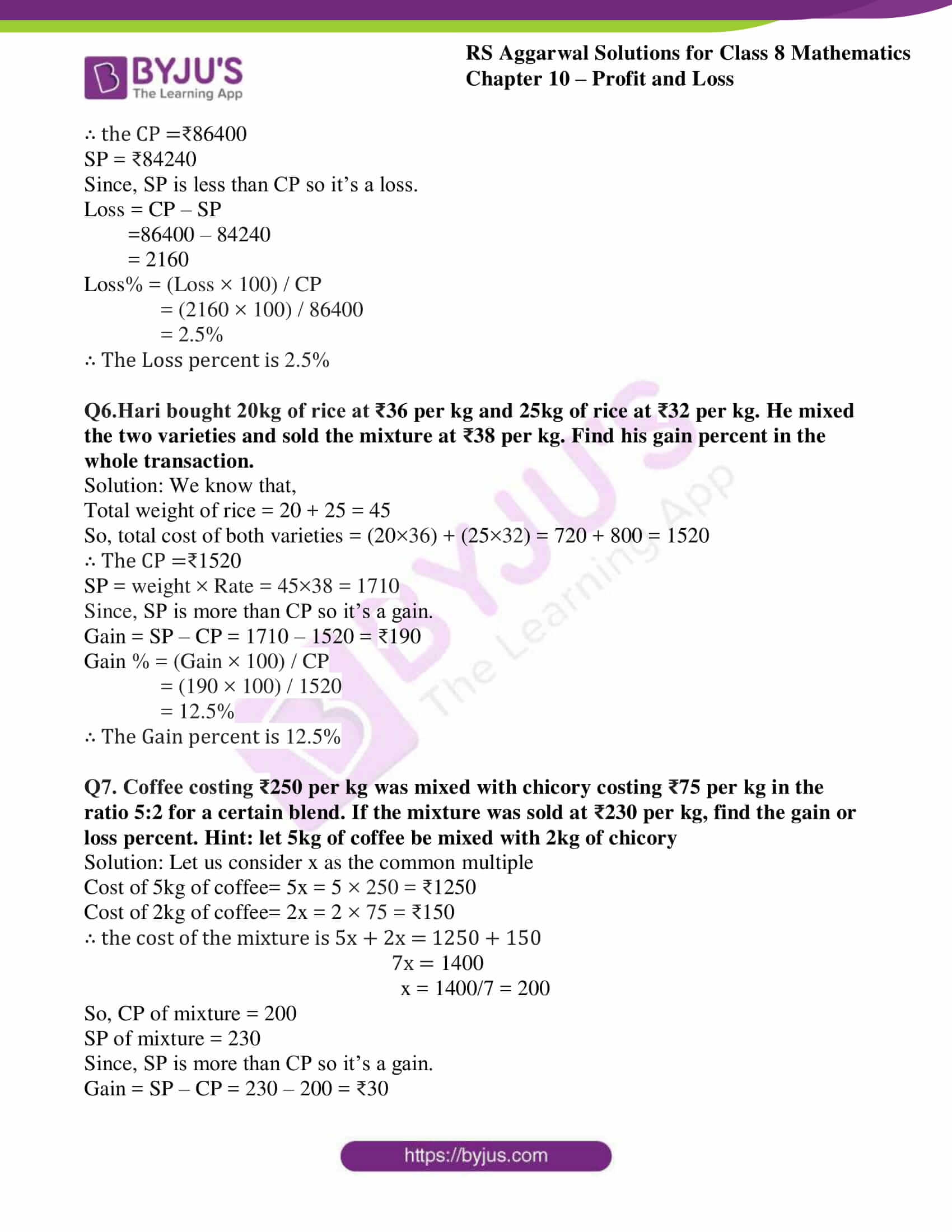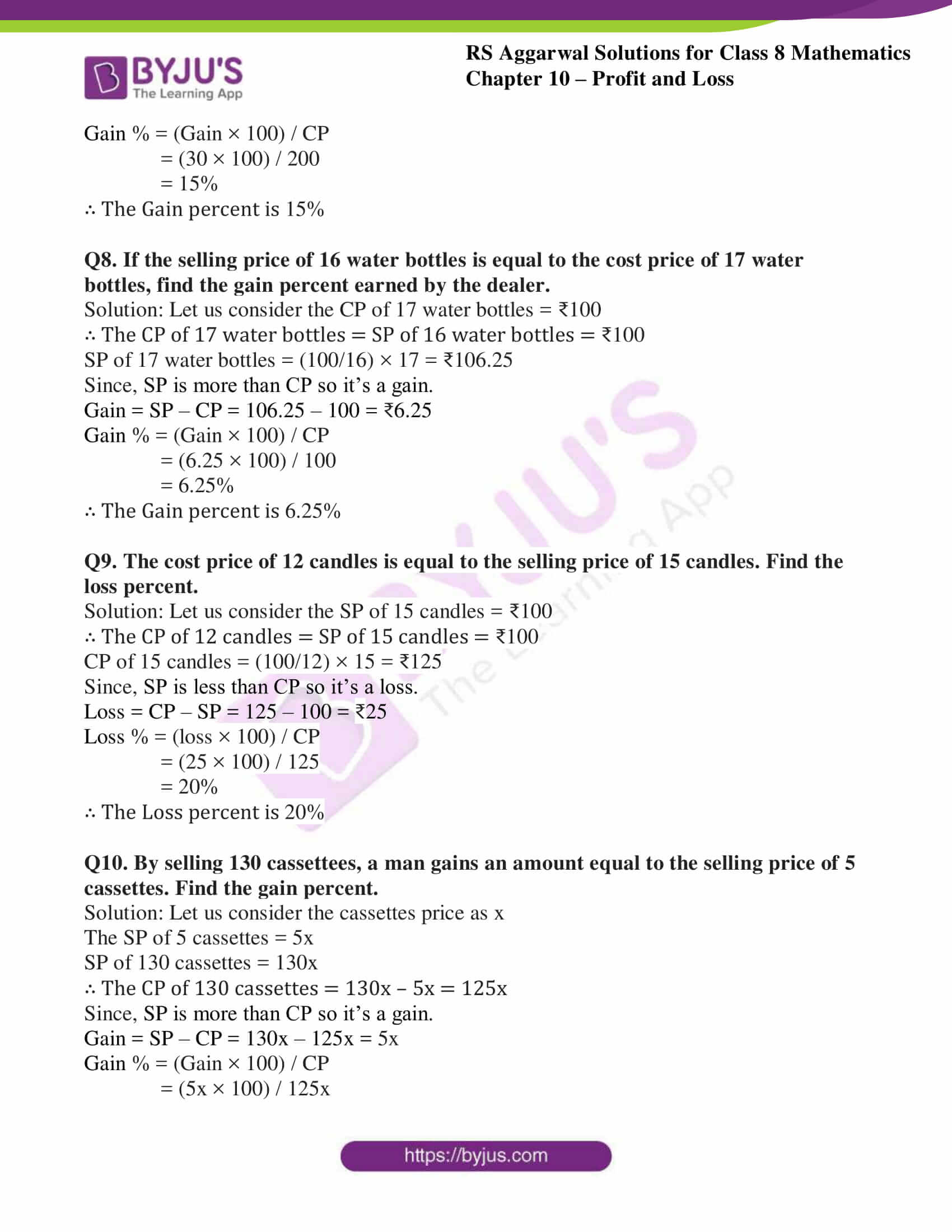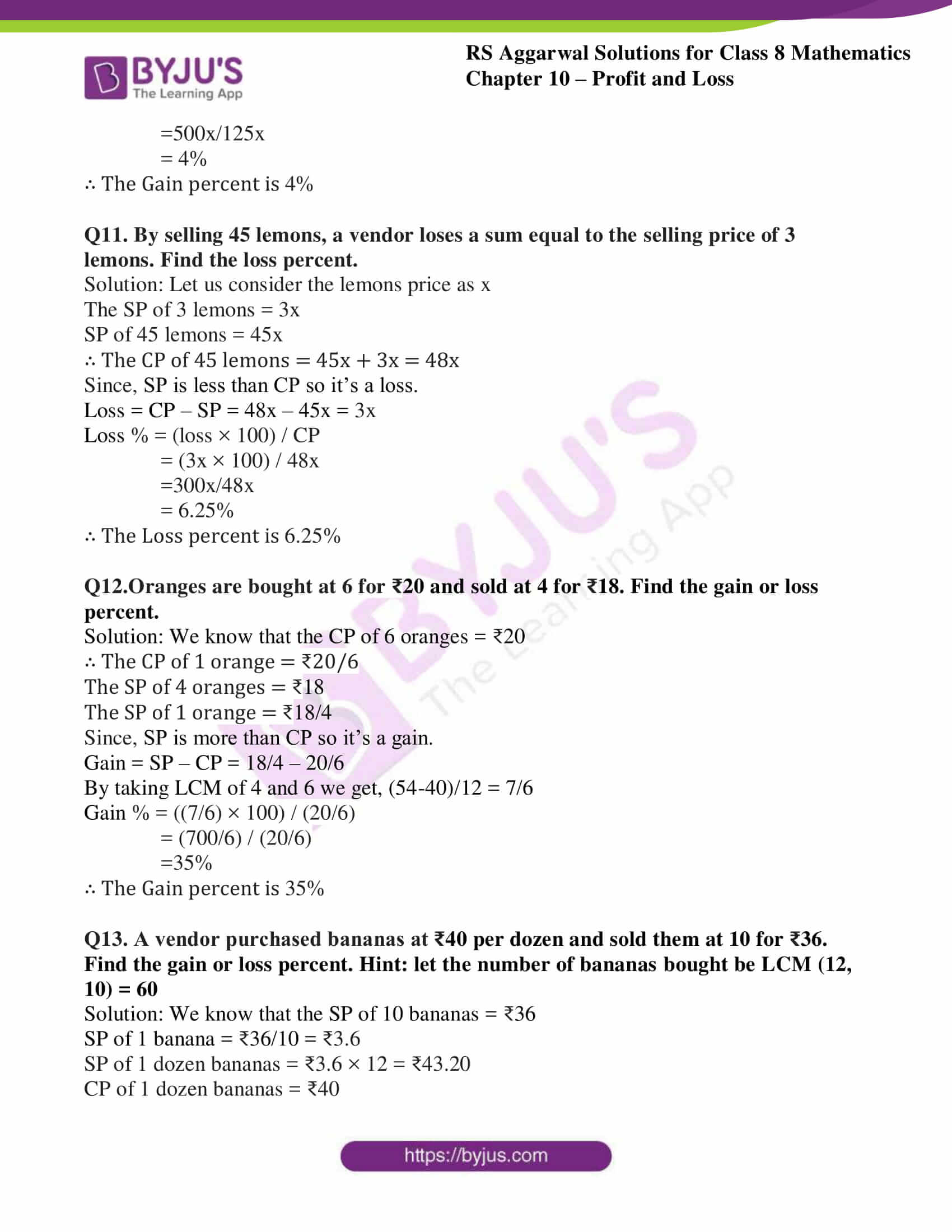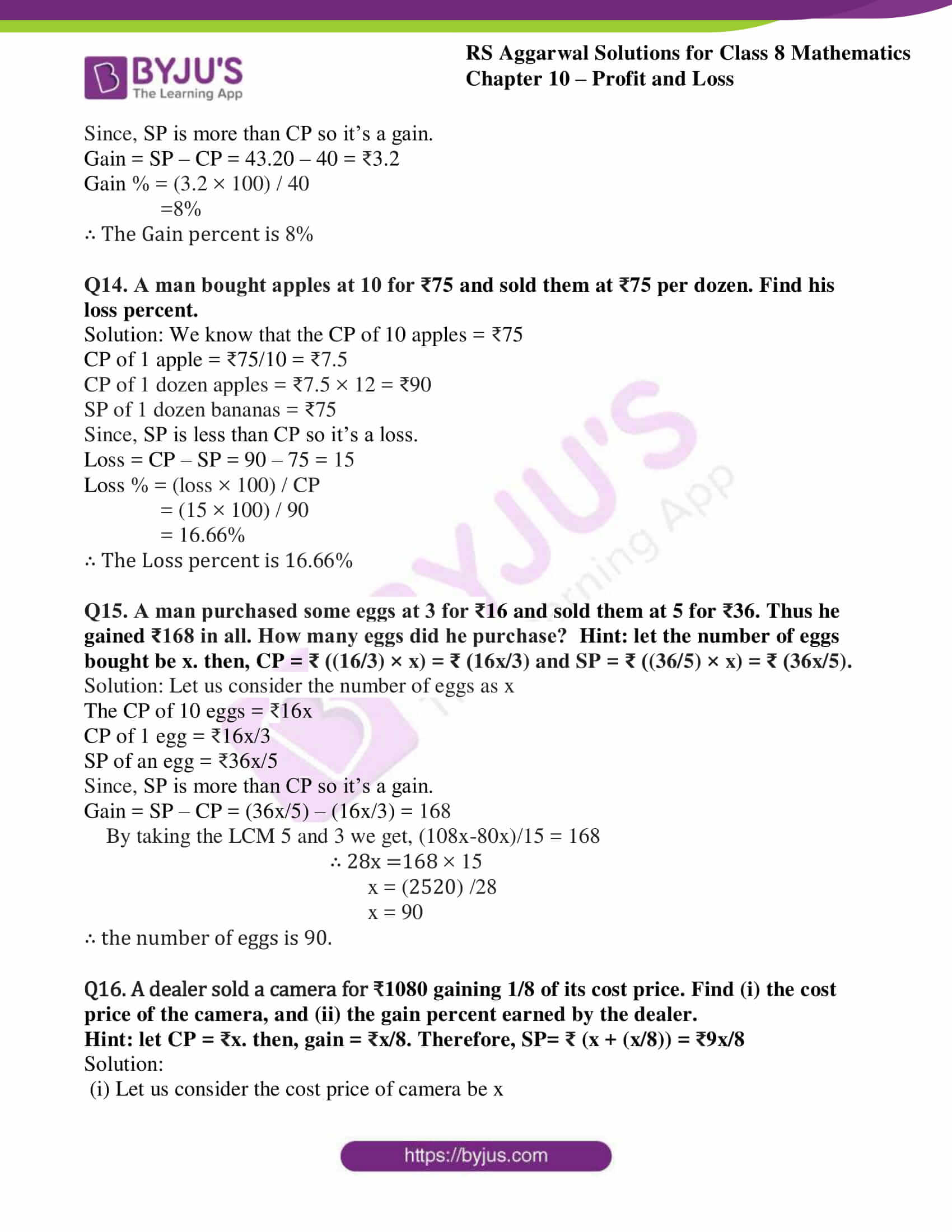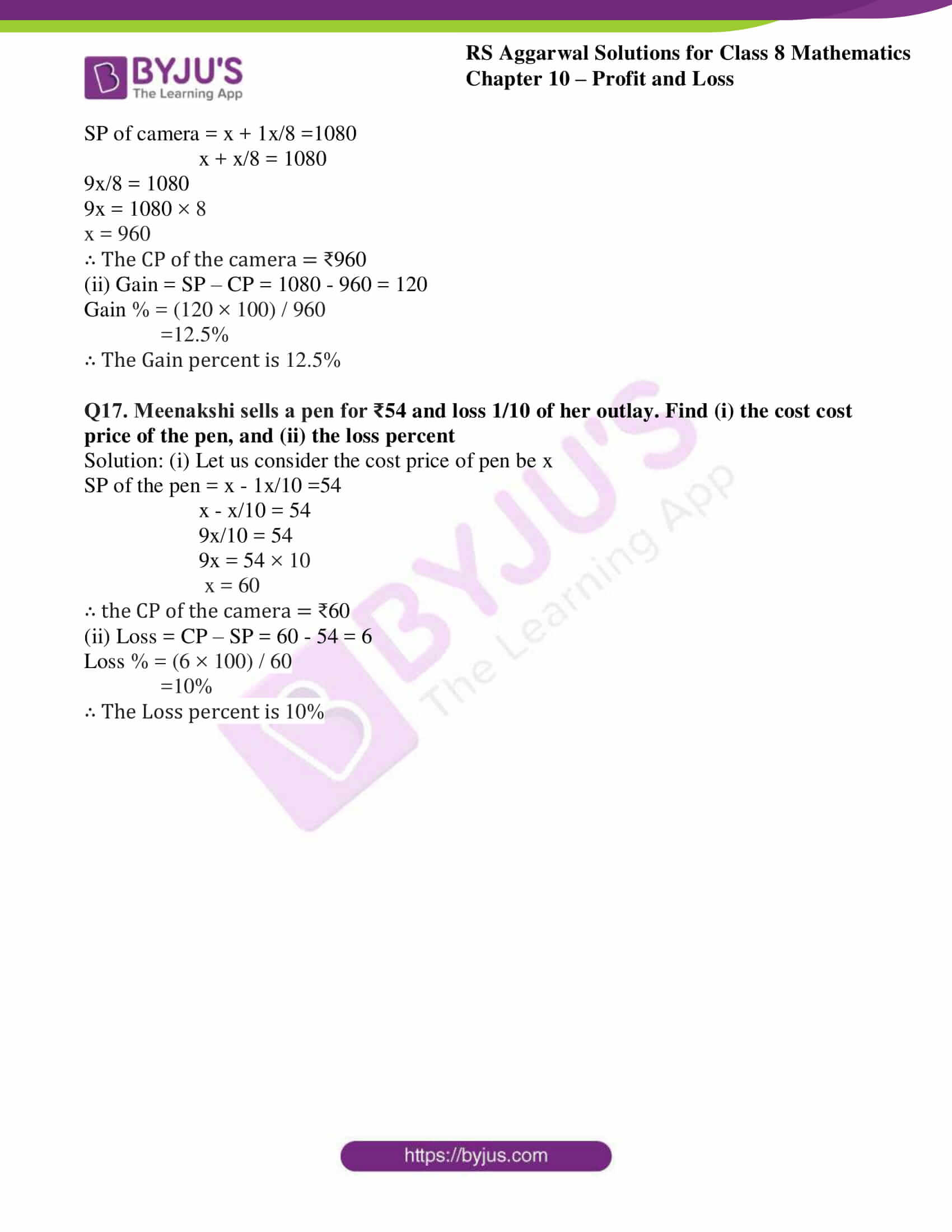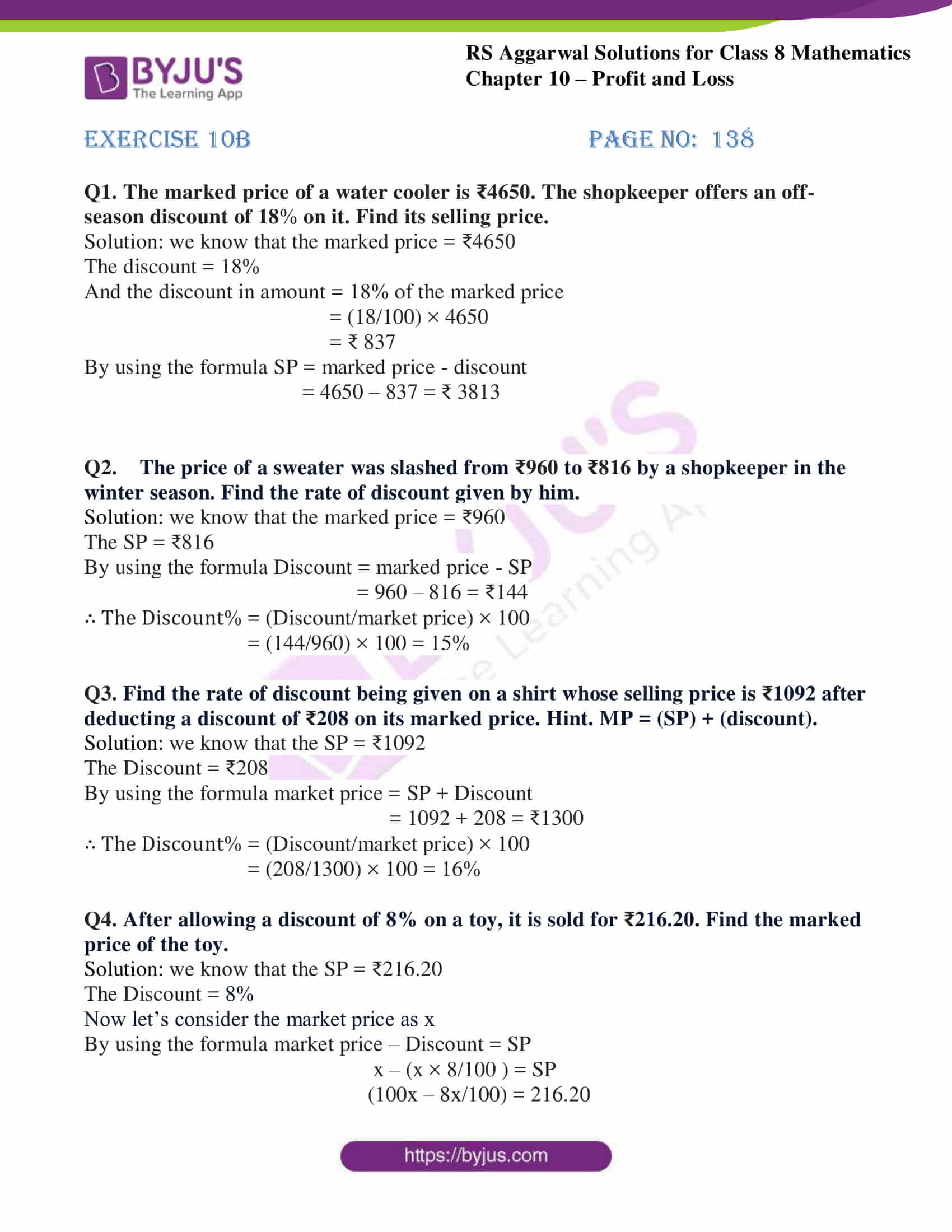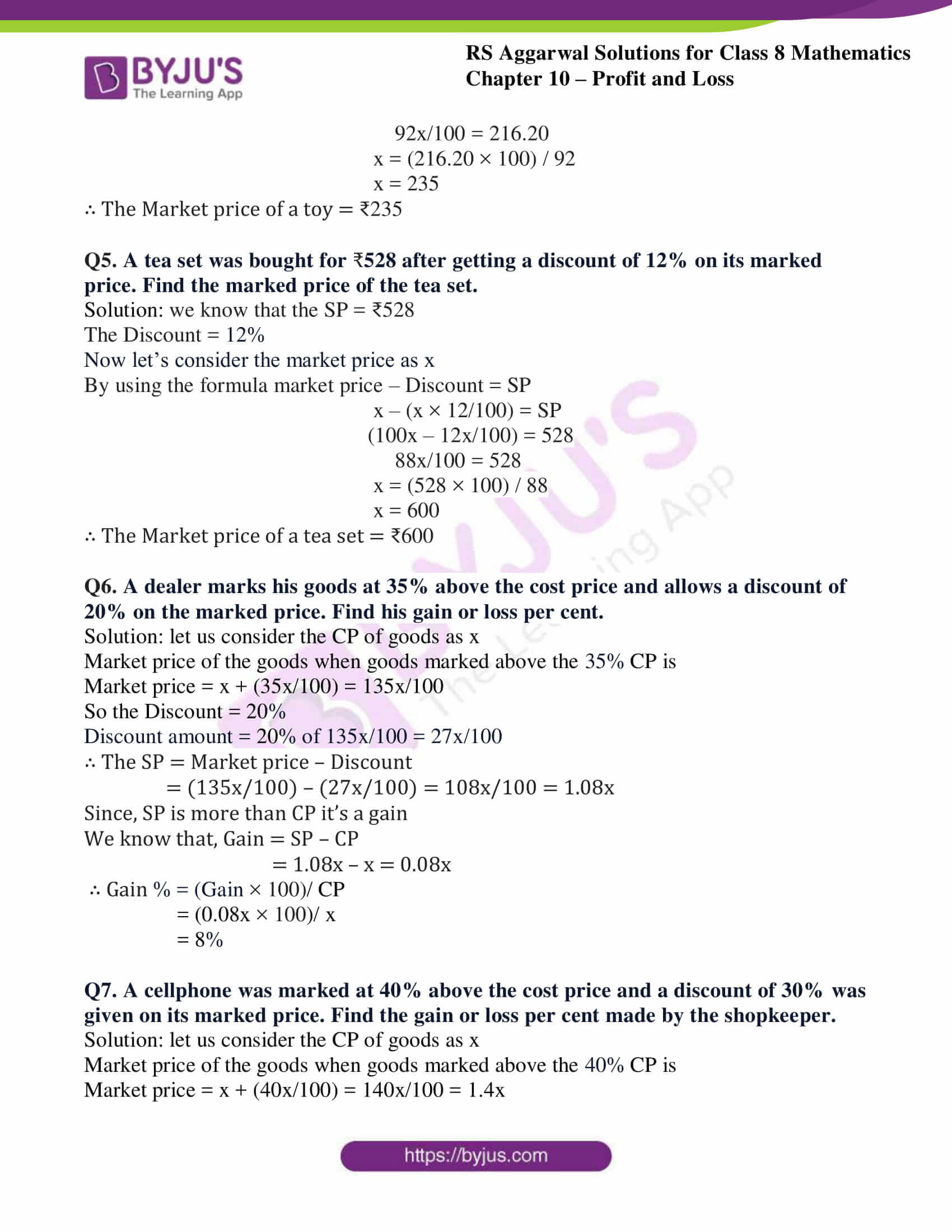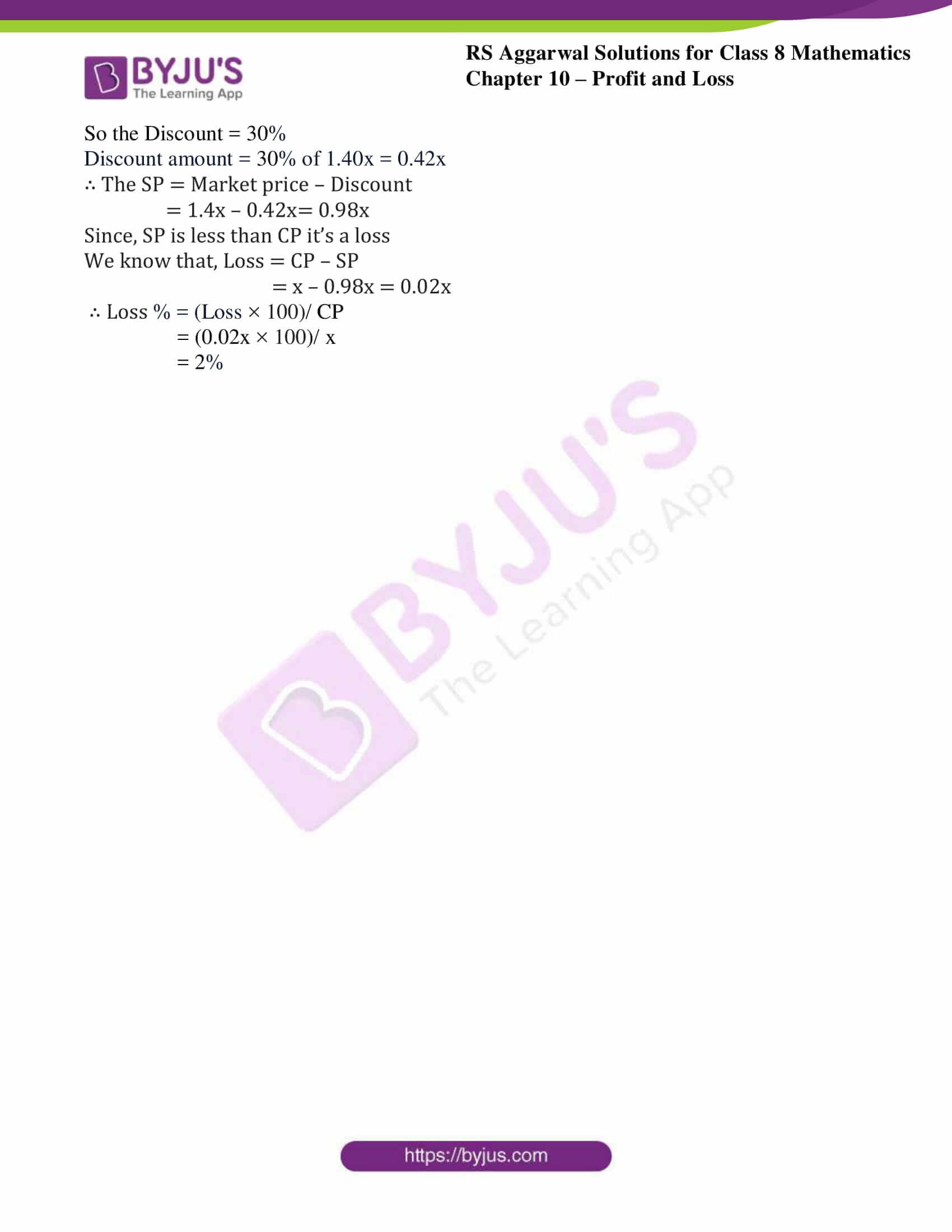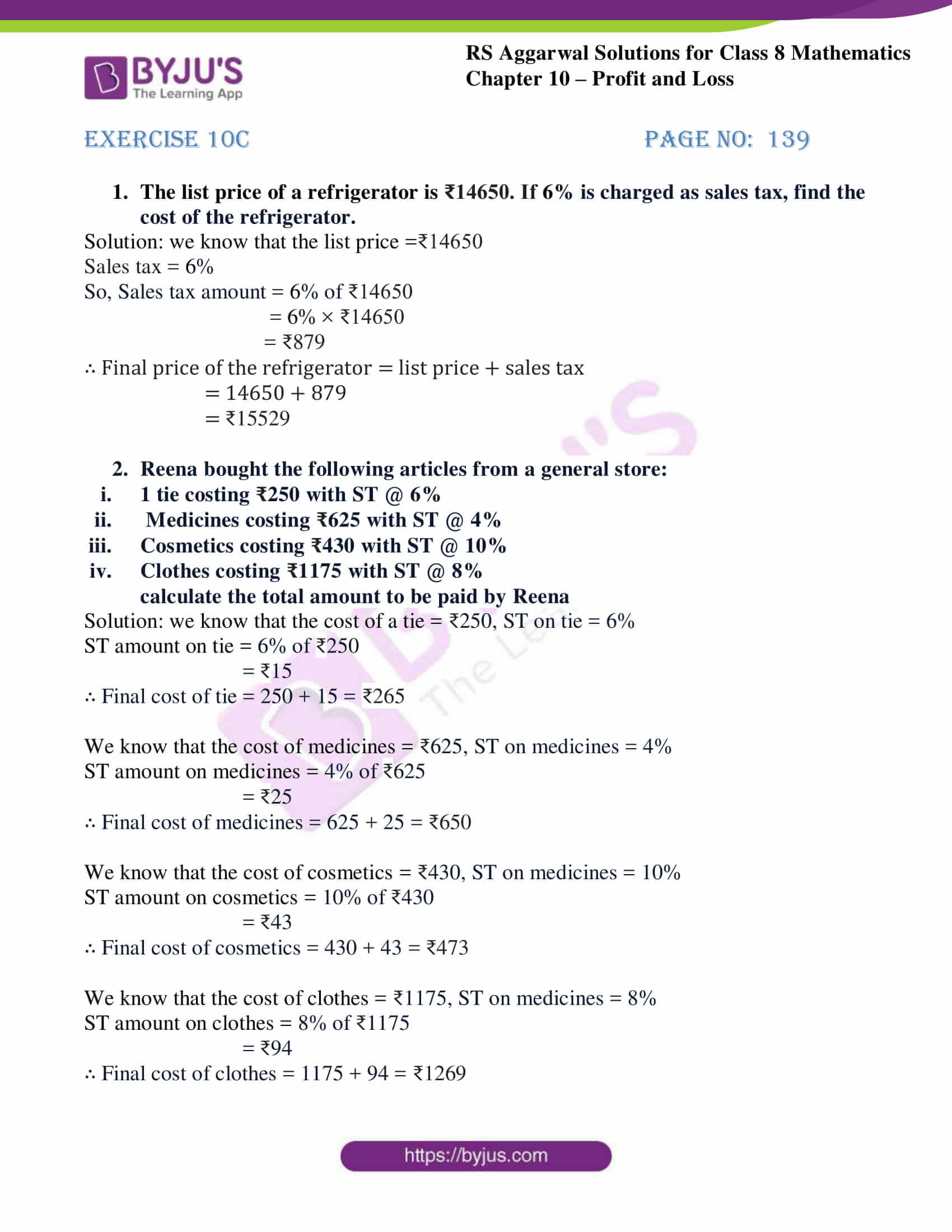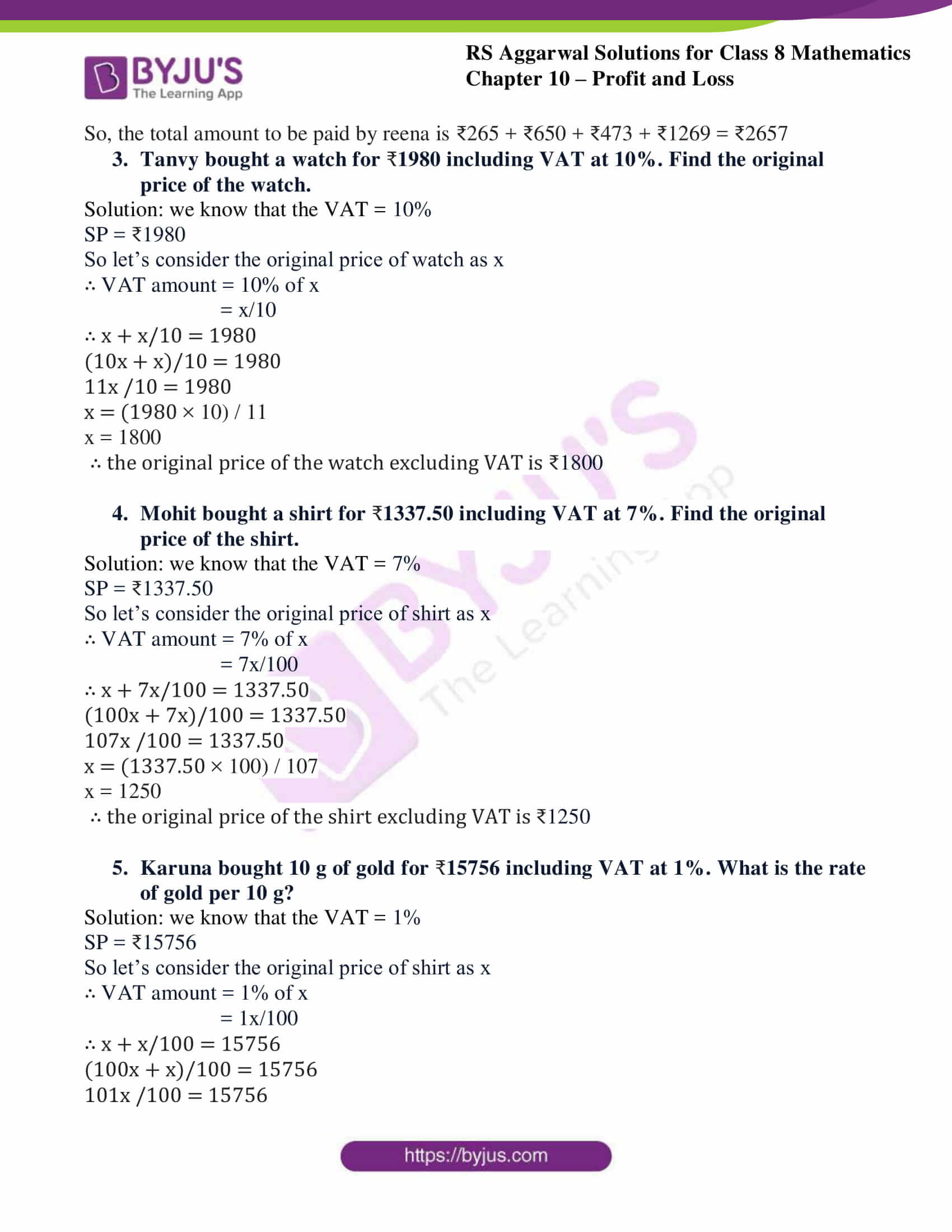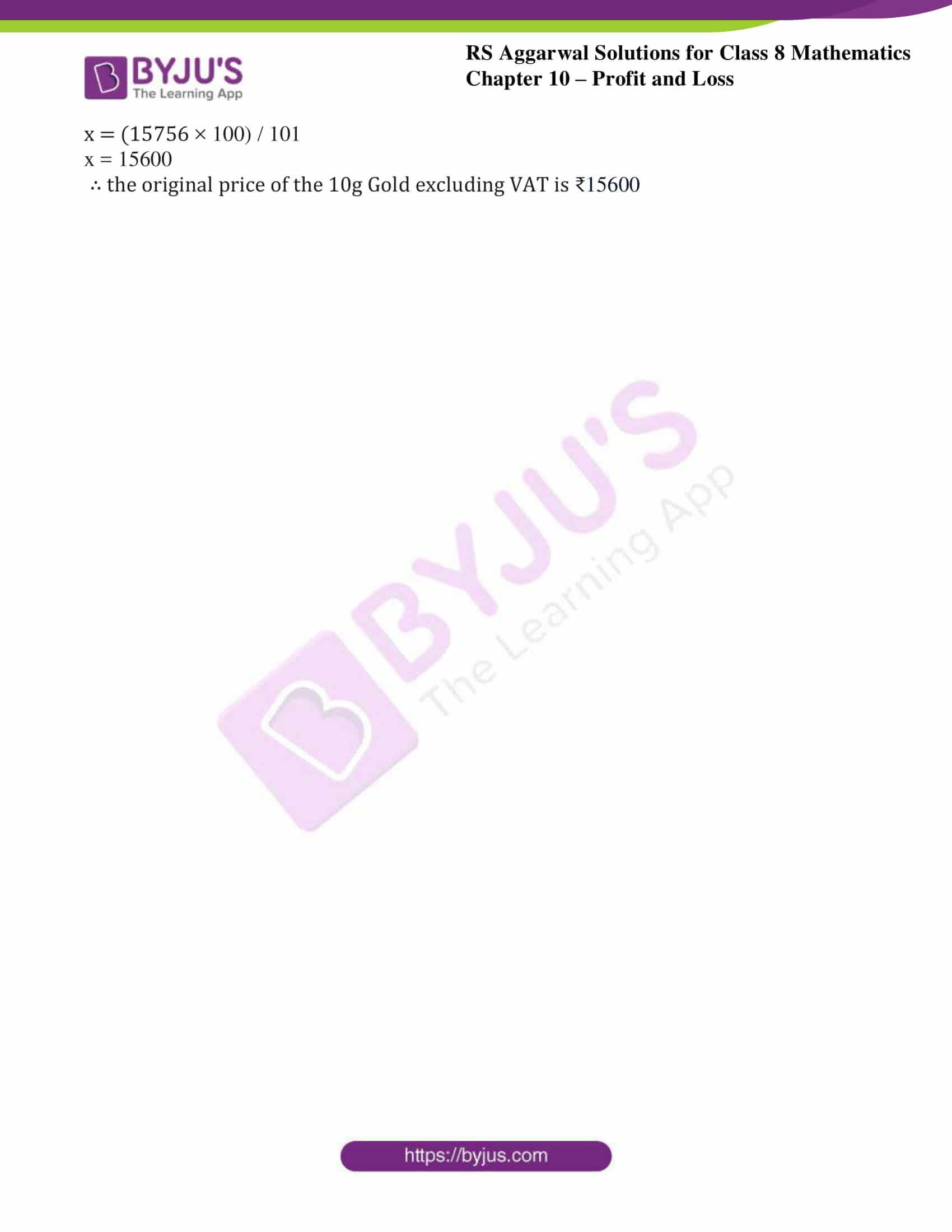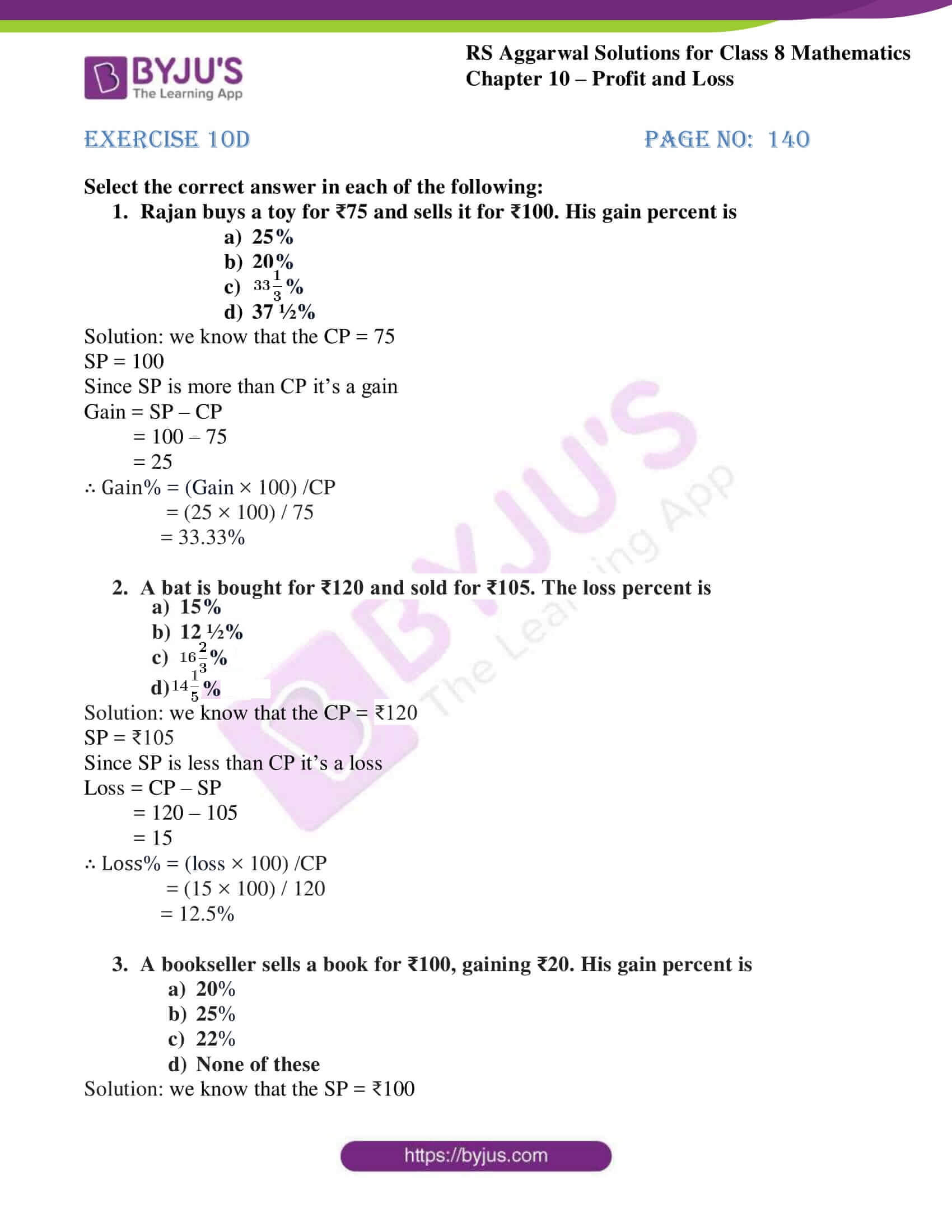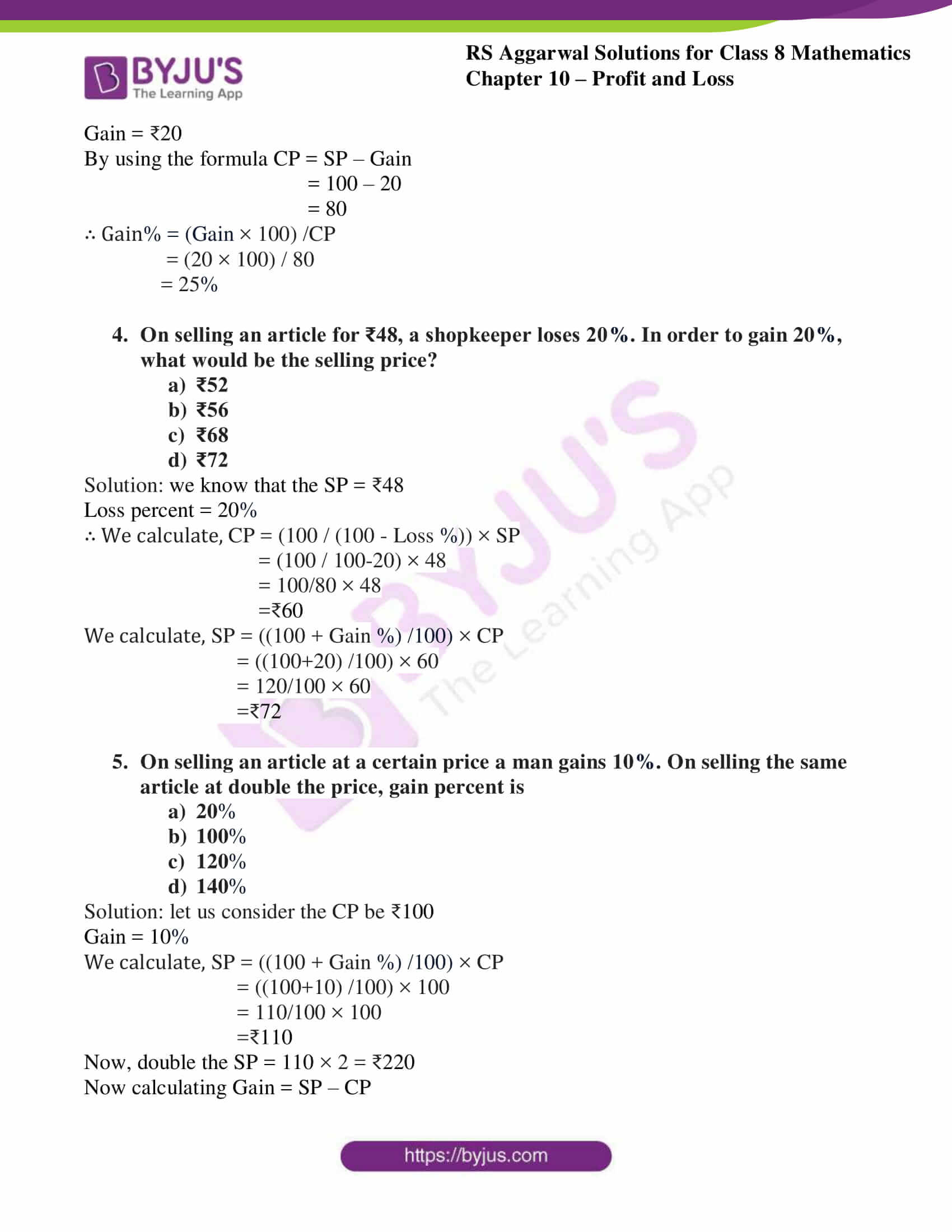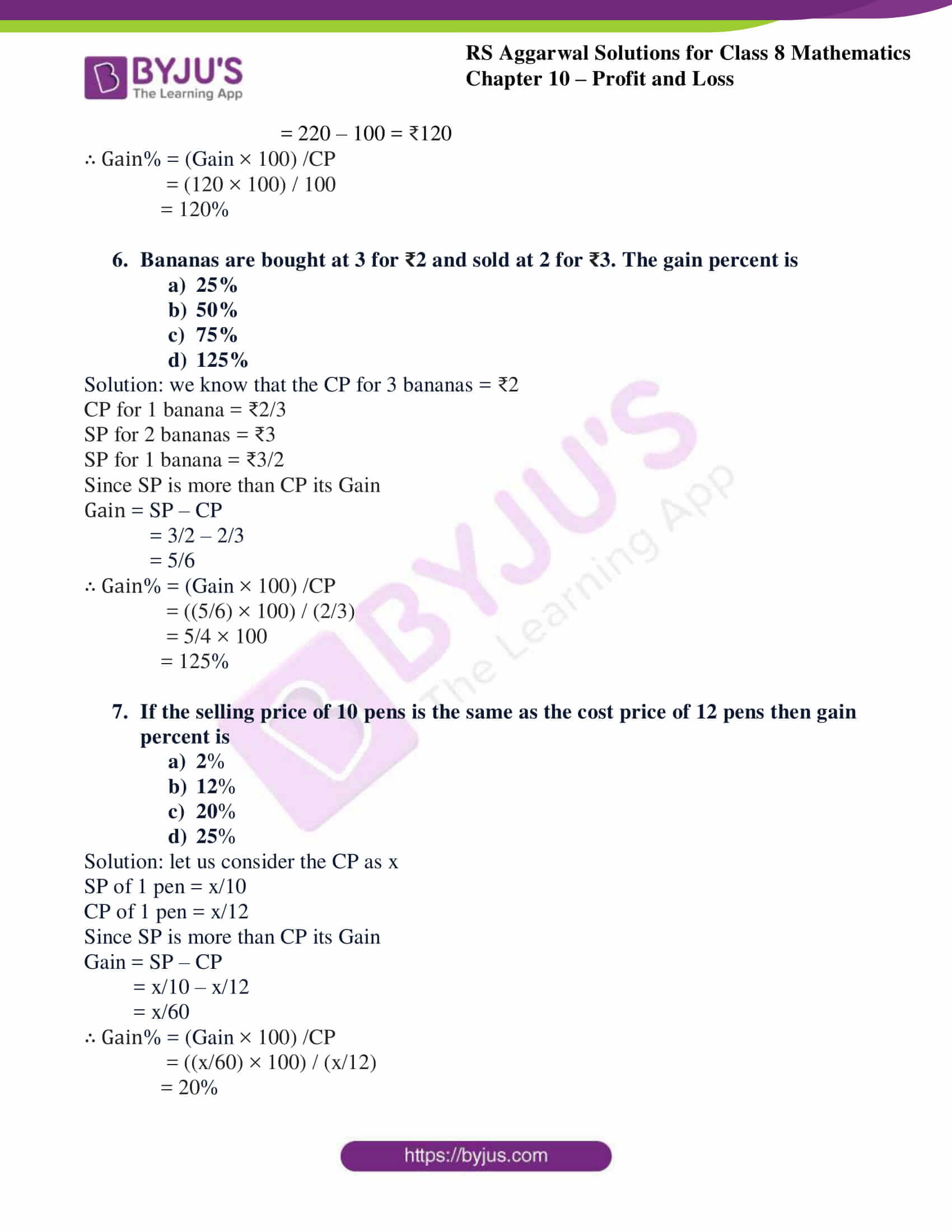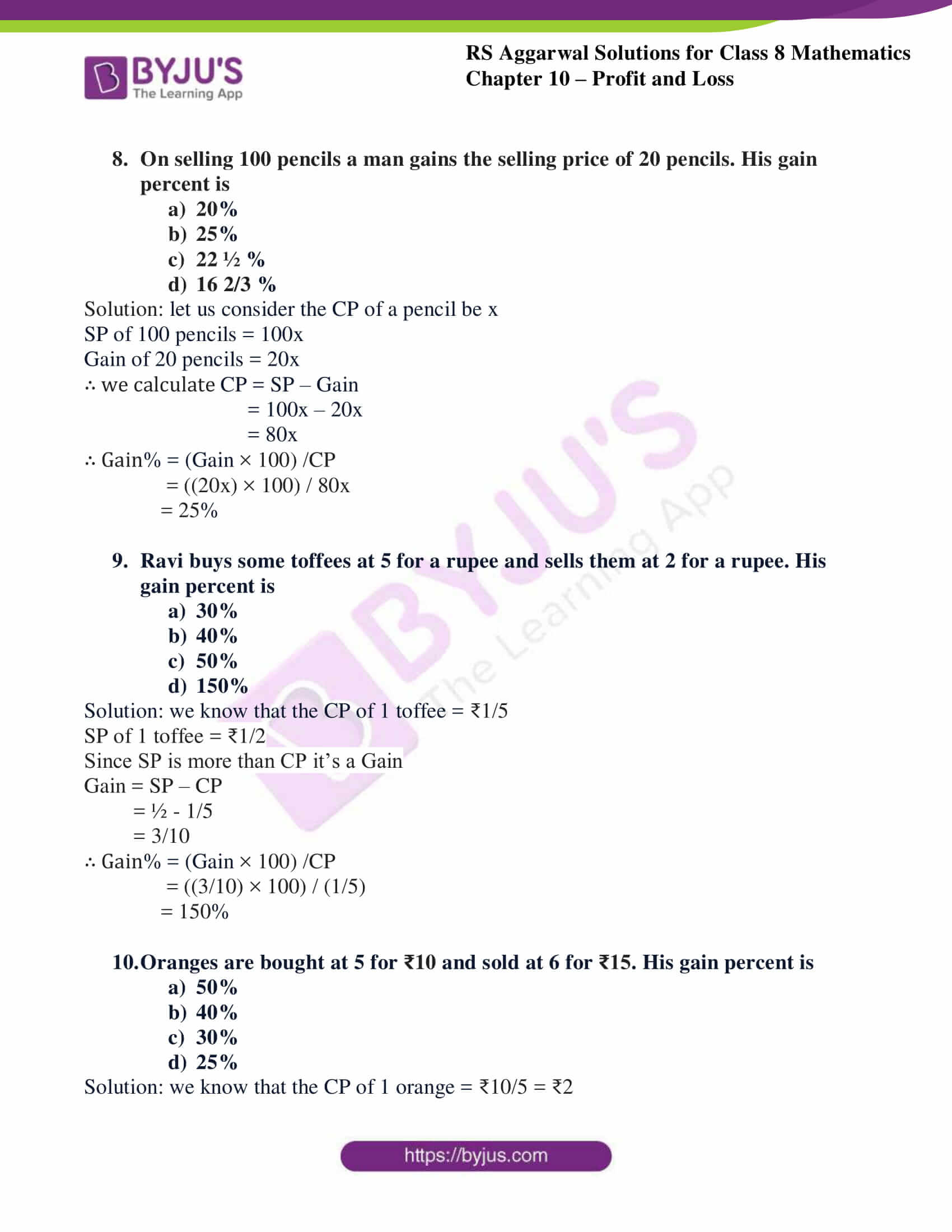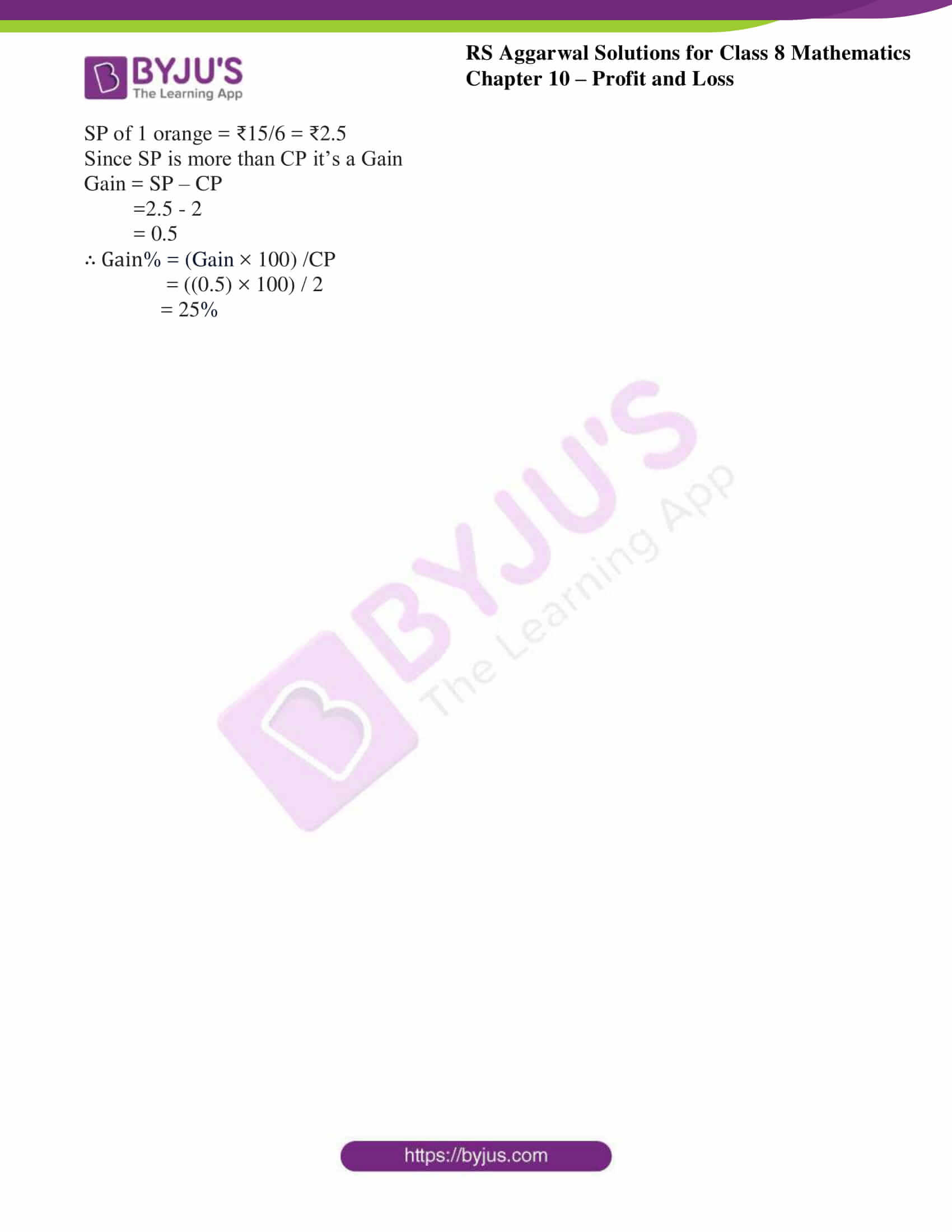## Exercise 10A

Q1.Find the gain or loss percent when:

i CP = ₹620 and SP = ₹713

Solution: we know that SP is more than CP so it’s a gain.As the formula states Gain = SP – CP713 – 620 = 93

Gain% = (Gain × 100) / CP

= (93 × 100) / 620

= 15%

ii CP = ₹675 and SP = ₹630

Solution: we know that SP is less than CP so it’s a loss.As the formula states Loss = CP – SP675 – 630 = 45

Loss% = (Loss × 100) / CP

= (45 × 100) / 675

= 6.66%

iii CP = ₹345 and SP = ₹372.60

Solution: we know that SP is more than CP so it’s a gain.As the formula states Gain = SP – CP372.60 – 345 = 27.60

Gain% = (Gain × 100) / CP

= (27.60 × 100) / 345

= 8%

iv CP = ₹80 and SP = ₹76.80

Solution: we know that SP is less than CP so it’s a loss.As the formula states Loss = CP – SP80 – 76.80 = 3.20

Loss% = (Loss × 100) / CP

= (3.20 × 100) / 80

= 4%

Q2.Find the selling price when:

i CP = ₹1650 and Gain = 4%

Solution: As the formula states SP = ((100 + Gain %) /100) × CP= ((100+4)/100) × 1650= (104/100) × 1650

=1716

∴ The selling price is 1716

ii CP = ₹915 and Gain = $\mathbf{6\frac{2}{3}}$%

Solution: As the formula states SP = ((100 + Gain %) /100) × CP= ((100+(20/3))/100) × 915= ((320/3)/100) × 915

=976

∴ The selling price is 976

iii CP = ₹875 and Loss = 12%

Solution: As the formula states SP = ((100 – Loss %) /100) × CP= ((100 – 12)/100) × 875= (88/100) × 875

=770

∴ The selling price is 770

iv CP = ₹645 and Loss = $\mathbf{13\frac{1}{3}}$%

Solution: As the formula states SP = ((100 – Loss %) /100) × CP= ((100-(40/3))/100) × 645= ((260/3)/100) × 645

= (260/300) × 645

=559

∴ The selling price is 559

Q3.Find the cost price when:

i SP = ₹1596 and Gain = 12%

Solution: As the formula states CP = (100 / (100+ Gain %)) × SP= (100/ (100+12)) × 1596= (100/112) × 1596

=1425

∴ The cost price is 1425

ii SP = ₹2431 and Loss = 6 ½ %

Solution: As the formula states CP = (100 / (100 – Loss %)) × SP= (100/ (100-(13/2))) × 2431= (100/ (187/2)) × 2431

= (200/187) × 2431

=2600

∴ The cost price is 2600

iii SP = ₹657.60 and Loss = 4%

Solution: As the formula states CP = (100 / (100 – Loss %)) × SP= (100/ (100-4)) × 657.60= (100/96) × 657.60

=658

∴ the cost price is 658

iv SP = ₹34.40 and Gain = 7 ½ %

Solution: As the formula states CP = (100 / (100+ Gain %)) × SP= (100/ (100+ (15/2))) × 34.40= (100/ (215/2)) × 34.40

= (200/215) × 34.40

=32

∴ the cost price is 32

Q4. Manjit bought an iron safe for ₹12160 and paid ₹340 for its transportation. Then, he sold it for ₹12875. Find his gain percent.

Solution: Lets solve by using, the total cost of iron safe = purchase cost + transportation

= 12160 + 340 = 12500

CP of iron safe = 12500

SP of iron safe = 12875

Since, SP is more than CP so it’s a gain.

Gain = SP – CP = 12875 – 12500 = 375

Gain % = (Gain × 100) / CP

= (375 × 100) / 12500

= 3%

∴ The Gain percent is 3%

Q5. Robin purchased an old car for ₹73500. He spent ₹10300 on repairs and paid ₹2600 for its insurance. Then he sold it to a mechanic for ₹84240. What was his percentage gain or loss? Hint: overheads =₹10300+₹2600= ₹12900

Solution: We know that,

Actual price of old car= purchase price + overheads

=73500 + 12900

=86400

∴ the CP =₹86400

SP = ₹84240

Since, SP is less than CP so it’s a loss.

Loss = CP – SP

=86400 – 84240

= 2160

Loss% = (Loss × 100) / CP

= (2160 × 100) / 86400

= 2.5%

∴ The Loss percent is 2.5%

Q6.Hari bought 20kg of rice at ₹36 per kg and 25kg of rice at ₹32 per kg. He mixed the two varieties and sold the mixture at ₹38 per kg. Find his gain percent in the whole transaction.

Solution: We know that,

Total weight of rice = 20 + 25 = 45

So, total cost of both varieties = (20×36) + (25×32) = 720 + 800 = 1520

∴ The CP =₹1520

SP = weight × Rate = 45×38 = 1710

Since, SP is more than CP so it’s a gain.

Gain = SP – CP = 1710 – 1520 = ₹190

Gain % = (Gain × 100) / CP

= (190 × 100) / 1520

= 12.5%

∴ The Gain percent is 12.5%

Q7. Coffee costing ₹250 per kg was mixed with chicory costing ₹75 per kg in the ratio 5:2 for a certain blend. If the mixture was sold at ₹230 per kg, find the gain or loss percent. Hint: let 5kg of coffee be mixed with 2kg of chicory

Solution: Let us consider x as the common multiple

Cost of 5kg of coffee= 5x = 5 × 250 = ₹1250

Cost of 2kg of coffee= 2x = 2 × 75 = ₹150

∴ the cost of the mixture is 5x + 2x = 1250 + 150

7x = 1400

x = 1400/7 = 200

So, CP of mixture = 200

SP of mixture = 230

Since, SP is more than CP so it’s a gain.

Gain = SP – CP = 230 – 200 = ₹30

Gain % = (Gain × 100) / CP

= (30 × 100) / 200

= 15%

∴ The Gain percent is 15%

Q8. If the selling price of 16 water bottles is equal to the cost price of 17 water bottles, find the gain percent earned by the dealer.

Solution: Let us consider the CP of 17 water bottles = ₹100

∴ The CP of 17 water bottles = SP of 16 water bottles = ₹100

SP of 17 water bottles = (100/16) × 17 = ₹106.25

Since, SP is more than CP so it’s a gain.

Gain = SP – CP = 106.25 – 100 = ₹6.25

Gain % = (Gain × 100) / CP

= (6.25 × 100) / 100

= 6.25%

∴ The Gain percent is 6.25%

Q9. The cost price of 12 candles is equal to the selling price of 15 candles. Find the loss percent.

Solution: Let us consider the SP of 15 candles = ₹100

∴ The CP of 12 candles = SP of 15 candles = ₹100

CP of 15 candles = (100/12) × 15 = ₹125

Since, SP is less than CP so it’s a loss.

Loss = CP – SP = 125 – 100 = ₹25

Loss % = (loss × 100) / CP

= (25 × 100) / 125

= 20%

∴ The Loss percent is 20%

Q10. By selling 130 cassettees, a man gains an amount equal to the selling price of 5 cassettes. Find the gain percent.

Solution: Let us consider the cassettes price as x

The SP of 5 cassettes = 5x

SP of 130 cassettes = 130x

∴ The CP of 130 cassettes = 130x – 5x = 125x

Since, SP is more than CP so it’s a gain.

Gain = SP – CP = 130x – 125x = 5x

Gain % = (Gain × 100) / CP

= (5x × 100) / 125x

=500x/125x

= 4%

∴ The Gain percent is 4%

Q11. By selling 45 lemons, a vendor loses a sum equal to the selling price of 3 lemons. Find the loss percent.

Solution: Let us consider the lemons price as x

The SP of 3 lemons = 3x

SP of 45 lemons = 45x

∴ The CP of 45 lemons = 45x + 3x = 48x

Since, SP is less than CP so it’s a loss.

Loss = CP – SP = 48x – 45x = 3x

Loss % = (loss × 100) / CP

= (3x × 100) / 48x

=300x/48x

= 6.25%

∴ The Loss percent is 6.25%

Q12.Oranges are bought at 6 for ₹20 and sold at 4 for ₹18. Find the gain or loss percent.

Solution: We know that the CP of 6 oranges = ₹20

∴ The CP of 1 orange = ₹20/6

The SP of 4 oranges = ₹18

The SP of 1 orange = ₹18/4

Since, SP is more than CP so it’s a gain.

Gain = SP – CP = 18/4 – 20/6

By taking LCM of 4 and 6 we get, (54-40)/12 = 7/6

Gain % = ((7/6) × 100) / (20/6)

= (700/6) / (20/6)

=35%

∴ The Gain percent is 35%

Q13. A vendor purchased bananas at ₹40 per dozen and sold them at 10 for ₹36. Find the gain or loss percent. Hint: let the number of bananas bought be LCM (12, 10) = 60

Solution: We know that the SP of 10 bananas = ₹36

SP of 1 banana = ₹36/10 = ₹3.6

SP of 1 dozen bananas = ₹3.6 × 12 = ₹43.20

CP of 1 dozen bananas = ₹40

Since, SP is more than CP so it’s a gain.

Gain = SP – CP = 43.20 – 40 = ₹3.2

Gain % = (3.2 × 100) / 40

=8%

∴ The Gain percent is 8%

Q14. A man bought apples at 10 for ₹75 and sold them at ₹75 per dozen. Find his loss percent.

Solution: We know that the CP of 10 apples = ₹75

CP of 1 apple = ₹75/10 = ₹7.5

CP of 1 dozen apples = ₹7.5 × 12 = ₹90

SP of 1 dozen bananas = ₹75

Since, SP is less than CP so it’s a loss.

Loss = CP – SP = 90 – 75 = 15

Loss % = (loss × 100) / CP

= (15 × 100) / 90

= 16.66%

∴ The Loss percent is 16.66%

Q15. A man purchased some eggs at 3 for ₹16 and sold them at 5 for ₹36. Thus he gained ₹168 in all. How many eggs did he purchase? Hint: let the number of eggs bought be x. then, CP = ₹ ((16/3) × x) = ₹ (16x/3) and SP = ₹ ((36/5) × x) = ₹ (36x/5).

Solution: Let us consider the number of eggs as x

The CP of 10 eggs = ₹16x

CP of 1 egg = ₹16x/3

SP of an egg = ₹36x/5

Since, SP is more than CP so it’s a gain.

Gain = SP – CP = (36x/5) – (16x/3) = 168

By taking the LCM 5 and 3 we get, (108x-80x)/15 = 168

∴ 28x =168 × 15

x = (2520) /28

x = 90

∴ the number of eggs is 90.

Q16. A dealer sold a camera for ₹1080 gaining 1/8 of its cost price. Find (i) the cost price of the camera, and (ii) the gain percent earned by the dealer.

Hint: let CP = ₹x. then, gain = ₹x/8. Therefore, SP= ₹ (x + (x/8)) = ₹9x/8

Solution:

(i) Let us consider the cost price of camera be x

SP of camera = x + 1x/8 =1080

x + x/8 = 1080

9x/8 = 1080

9x = 1080 × 8

x = 960

∴ The CP of the camera = ₹960

(ii) Gain = SP – CP = 1080 – 960 = 120

Gain % = (120 × 100) / 960

=12.5%

∴ The Gain percent is 12.5%

Q17. Meenakshi sells a pen for ₹54 and loss 1/10 of her outlay. Find (i) the cost cost price of the pen, and (ii) the loss percent

Solution: (i) Let us consider the cost price of pen be x

SP of the pen = x – 1x/10 =54

x – x/10 = 54

9x/10 = 54

9x = 54 × 10

x = 60

∴ the CP of the camera = ₹60

(ii) Loss = CP – SP = 60 – 54 = 6

Loss % = (6 × 100) / 60

=10%

∴ The Loss percent is 10%

## Exercise 10B

Q1. The marked price of a water cooler is ₹4650. The shopkeeper offers an off-season discount of 18% on it. Find its selling price.

Solution: we know that the marked price = ₹4650

The discount = 18%

And the discount in amount = 18% of the marked price

= (18/100) × 4650

= ₹ 837

By using the formula SP = marked price – discount

= 4650 – 837 = ₹ 3813

Q2. The price of a sweater was slashed from ₹960 to ₹816 by a shopkeeper in the winter season. Find the rate of discount given by him.

Solution: we know that the marked price = ₹960

The SP = ₹816

By using the formula Discount = marked price – SP

= 960 – 816 = ₹144

∴ The Discount% = (Discount/market price) × 100

= (144/960) × 100 = 15%

Q3. Find the rate of discount being given on a shirt whose selling price is ₹1092 after deducting a discount of ₹208 on its marked price. Hint. MP = (SP) + (discount).

Solution: we know that the SP = ₹1092

The Discount = ₹208

By using the formula market price = SP + Discount

= 1092 + 208 = ₹1300

∴ The Discount% = (Discount/market price) × 100

= (208/1300) × 100 = 16%

Q4. After allowing a discount of 8% on a toy, it is sold for ₹216.20. Find the marked price of the toy.

Solution: we know that the SP = ₹216.20

The Discount = 8%

Now let’s consider the market price as x

By using the formula market price – Discount = SP

x – (x × 8/100 ) = SP

(100x – 8x/100) = 216.20

92x/100 = 216.20

x = (216.20 × 100) / 92

x = 235

∴ The Market price of a toy = ₹235

Q5. A tea set was bought for 528 after getting a discount of 12% on its marked price. Find the marked price of the tea set.

Solution: we know that the SP = ₹528

The Discount = 12%

Now let’s consider the market price as x

By using the formula market price – Discount = SP

x – (x × 12/100) = SP

(100x – 12x/100) = 528

88x/100 = 528

x = (528 × 100) / 88

x = 600

∴ The Market price of a tea set = ₹600

Q6. A dealer marks his goods at 35% above the cost price and allows a discount of 20% on the marked price. Find his gain or loss per cent.

Solution: let us consider the CP of goods as x

Market price of the goods when goods marked above the 35% CP is

Market price = x + (35x/100) = 135x/100

So the Discount = 20%

Discount amount = 20% of 135x/100 = 27x/100

∴ The SP = Market price – Discount

= (135x/100) – (27x/100) = 108x/100 = 1.08x

Since, SP is more than CP it’s a gain

We know that, Gain = SP – CP

= 1.08x – x = 0.08x

∴ Gain % = (Gain × 100)/ CP

= (0.08x × 100)/ x

= 8%

Q7. A cellphone was marked at 40% above the cost price and a discount of 30% was given on its marked price. Find the gain or loss per cent made by the shopkeeper.

Solution: let us consider the CP of goods as x

Market price of the goods when goods marked above the 40% CP is

Market price = x + (40x/100) = 140x/100 = 1.4x

So the Discount = 30%

Discount amount = 30% of 1.40x = 0.42x

∴ The SP = Market price – Discount

= 1.4x – 0.42x= 0.98x

Since, SP is less than CP it’s a loss

We know that, Loss = CP – SP

= x – 0.98x = 0.02x

∴ Loss % = (Loss × 100)/ CP

= (0.02x × 100)/ x

= 2%

## Exercise 10C

Q1. The list price of a refrigerator is ₹14650. If 6% is charged as sales tax, find the cost of the refrigerator.

Solution: we know that the list price =₹14650

Sales tax = 6%

So, Sales tax amount = 6% of ₹14650

= 6% × ₹14650

= ₹879

∴ Final price of the refrigerator = list price + sales tax

= 14650 + 879

= ₹15529

Q2. Reena bought the following articles from a general store:

i. 1 tie costing ₹250 with ST @ 6%
ii. Medicines costing ₹625 with ST @ 4%
iii. Cosmetics costing ₹430 with ST @ 10%
iv. Clothes costing ₹1175 with ST @ 8%
calculate the total amount to be paid by Reena

Solution: we know that the cost of a tie = ₹250, ST on tie = 6%

ST amount on tie = 6% of ₹250

= ₹15

∴ Final cost of tie = 250 + 15 = ₹265

We know that the cost of medicines = ₹625, ST on medicines = 4%

ST amount on medicines = 4% of ₹625

= ₹25

∴ Final cost of medicines = 625 + 25 = ₹650

We know that the cost of cosmetics = ₹430, ST on medicines = 10%

ST amount on cosmetics = 10% of ₹430

= ₹43

∴ Final cost of cosmetics = 430 + 43 = ₹473

We know that the cost of clothes = ₹1175, ST on medicines = 8%

ST amount on clothes = 8% of ₹1175

= ₹94

∴ Final cost of clothes = 1175 + 94 = ₹1269

So, the total amount to be paid by reena is ₹265 + ₹650 + ₹473 + ₹1269 = ₹2657

Q3. Tanvy bought a watch for 1980 including VAT at 10%. Find the original price of the watch.

Solution: we know that the VAT = 10%

SP = ₹1980

So let’s consider the original price of watch as x

∴ VAT amount = 10% of x

= x/10

∴ x + x/10 = 1980

(10x + x)/10 = 1980

11x /10 = 1980

x = (1980 × 10) / 11

x = 1800

∴ the original price of the watch excluding VAT is ₹1800

Q4. Mohit bought a shirt for 1337.50 including VAT at 7%. Find the original price of the shirt.

Solution: we know that the VAT = 7%

SP = ₹1337.50

So let’s consider the original price of shirt as x

∴ VAT amount = 7% of x

= 7x/100

∴ x + 7x/100 = 1337.50

(100x + 7x)/100 = 1337.50

107x /100 = 1337.50

x = (1337.50 × 100) / 107

x = 1250

∴ the original price of the shirt excluding VAT is ₹1250

Q5. Karuna bought 10 g of gold for 15756 including VAT at 1%. What is the rate of gold per 10 g?

Solution: we know that the VAT = 1%

SP = ₹15756

So let’s consider the original price of shirt as x

∴ VAT amount = 1% of x

= 1x/100

∴ x + x/100 = 15756

(100x + x)/100 = 15756

101x /100 = 15756

x = (15756 × 100) / 101

x = 15600

∴ the original price of the 10g Gold excluding VAT is ₹15600

## Exercise 10D

Select the correct answer in each of the following:

1. Rajan buys a toy for ₹75 and sells it for ₹100. His gain percent is

1. 25%
2. 20%
3. $33\frac{1}{3}$%
4. 37 ½%

Solution: we know that the CP = 75

SP = 100

Since SP is more than CP it’s a gain

Gain = SP – CP

= 100 – 75

= 25

∴ Gain% = (Gain × 100) /CP

= (25 × 100) / 75

= 33.33%

2. A bat is bought for ₹120 and sold for ₹105. The loss percent is

1. 15%
2. 12 ½%
3. $16\frac{2}{3}$%
4. $14\frac{1}{5}$%

Solution: we know that the CP = ₹120

SP = ₹105

Since SP is less than CP it’s a loss

Loss = CP – SP

= 120 – 105

= 15

∴ Loss% = (loss × 100) /CP

= (15 × 100) / 120

= 12.5%

3. A bookseller sells a book for ₹100, gaining ₹20. His gain percent is

1. 20%
2. 25%
3. 22%
4. None of these

Solution: we know that the SP = ₹100

Gain = ₹20

By using the formula CP = SP – Gain

= 100 – 20

= 80

∴ Gain% = (Gain × 100) /CP

= (20 × 100) / 80

= 25%

4. On selling an article for ₹48, a shopkeeper loses 20%. In order to gain 20%, what would be the selling price?

1. ₹52
2. ₹56
3. ₹68
4. ₹72

Solution: we know that the SP = ₹48

Loss percent = 20%

∴ We calculate, CP = (100 / (100 – Loss %)) × SP

= (100 / 100-20) × 48

= 100/80 × 48

=₹60

We calculate, SP = ((100 + Gain %) /100) × CP

= ((100+20) /100) × 60

= 120/100 × 60

=₹72

5. On selling an article at a certain price a man gains 10%. On selling the same article at double the price, gain percent is

1. 20%
2. 100%
3. 120%
4. 140%

Solution: let us consider the CP be ₹100

Gain = 10%

We calculate, SP = ((100 + Gain %) /100) × CP

= ((100+10) /100) × 100

= 110/100 × 100

=₹110

Now, double the SP = 110 × 2 = ₹220

Now calculating Gain = SP – CP

= 220 – 100 = ₹120

∴ Gain% = (Gain × 100) /CP

= (120 × 100) / 100

= 120%

6. Bananas are bought at 3 for ₹2 and sold at 2 for ₹3. The gain percent is

1. 25%
2. 50%
3. 75%
4. 125%

Solution: we know that the CP for 3 bananas = ₹2

CP for 1 banana = ₹2/3

SP for 2 bananas = ₹3

SP for 1 banana = ₹3/2

Since SP is more than CP its Gain

Gain = SP – CP

= 3/2 – 2/3

= 5/6

∴ Gain% = (Gain × 100) /CP

= ((5/6) × 100) / (2/3)

= 5/4 × 100

= 125%

7. If the selling price of 10 pens is the same as the cost price of 12 pens then gain percent is

1. 2%
2. 12%
3. 20%
4. 25%

Solution: let us consider the CP as x

SP of 1 pen = x/10

CP of 1 pen = x/12

Since SP is more than CP its Gain

Gain = SP – CP

= x/10 – x/12

= x/60

∴ Gain% = (Gain × 100) /CP

= ((x/60) × 100) / (x/12)

= 20%

8. On selling 100 pencils a man gains the selling price of 20 pencils. His gain percent is

1. 20%
2. 25%
3. 22 ½ %
4. 16 2/3 %

Solution: let us consider the CP of a pencil be x

SP of 100 pencils = 100x

Gain of 20 pencils = 20x

∴ we calculate CP = SP – Gain

= 100x – 20x

= 80x

∴ Gain% = (Gain × 100) /CP

= ((20x) × 100) / 80x

= 25%

9. Ravi buys some toffees at 5 for a rupee and sells them at 2 for a rupee. His gain percent is

1. 30%
2. 40%
3. 50%
4. 150%

Solution: we know that the CP of 1 toffee = ₹1/5

SP of 1 toffee = ₹1/2

Since SP is more than CP it’s a Gain

Gain = SP – CP

= ½ – 1/5

= 3/10

∴ Gain% = (Gain × 100) /CP

= ((3/10) × 100) / (1/5)

= 150%

10. Oranges are bought at 5 for ₹10 and sold at 6 for ₹15. His gain percent is

1. 50%
2. 40%
3. 30%
4. 25%

Solution: we know that the CP of 1 orange = ₹10/5 = ₹2

SP of 1 orange = ₹15/6 = ₹2.5

Since SP is more than CP it’s a Gain

Gain = SP – CP

=2.5 – 2

= 0.5

∴ Gain% = (Gain × 100) /CP

= ((0.5) × 100) / 2

= 25%

## RS Aggarwal Solutions for Class 8 Maths Chapter 10 – Profit and Loss

Chapter 10- Profit and Loss contain four exercises and the RS Aggarwal solutions present on this page provide the solutions for the questions present in each exercise. Now, let us have a look at the concepts discussed in this chapter.

• Calculating the Cost price.
• Calculating the Selling price.
• Calculating the Profit or Gain ％.
• Calculating the Loss or Loss％.
• Calculating the Discount.
• Calculating the Sales Tax or Value added Tax(VAT).

Exercise 10A

Exercise 10B

Exercise 10C

Exercise 10D

### Chapter Brief of RS Aggarwal Solutions for Class 8 Maths Chapter 10 – Profit and Loss

RS Aggarwal Solutions for Class 8 Maths Chapter 10 – Profit and Loss, ensures that the students are thorough and familiar with the concepts. Regular revision of important concepts and formulas over time to time is the best way to strengthen your ability to solve the problems. The solutions are designed in such a way that they are easy to understand and solve.

As the Chapter is about Profit and Loss, now let us understand the concept of profit and loss in our daily life, as and when we purchase goods we generally use the statements such as cost price, selling price, profit, loss, and discount. These statements are financial statements that summarise the revenues, cost and expenses incurred during a specified period, usually a fiscal quarter or a year. Here, in the RS Aggarwal Solutions book, many such exercise problems are given which enhances familiarity with the concepts.